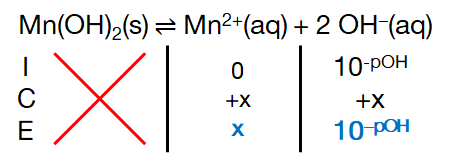# Problem: Calculate the solubility of Mn (OH)2 in grams per liter when buffered at pH (a) 7.0 (b) 9.5 (c) 11.8

###### FREE Expert Solution

Given: Mn(OH)2(s) ⇌ Mn2+(aq) + 2 OH(aq); Ksp = 1.6 × 10–13

Ksp Expression:

$\overline{){{\mathbf{K}}}_{{\mathbf{sp}}}{\mathbf{=}}\mathbf{\left[}{\mathbf{Mn}}^{\mathbf{2}\mathbf{+}}\mathbf{\right]}{\mathbf{\left[}{\mathbf{OH}}^{\mathbf{-}}\mathbf{\right]}}^{{\mathbf{2}}}}$

ICE Chart:Recall:

$\overline{){\mathbf{\left[}}{{\mathbf{OH}}}^{{\mathbf{-}}}{\mathbf{\right]}}{\mathbf{=}}{{\mathbf{10}}}^{\mathbf{-}\mathbf{pOH}}}\phantom{\rule{0ex}{0ex}}\overline{){\mathbf{pOH}}{\mathbf{=}}{\mathbf{14}}{\mathbf{-}}{\mathbf{pH}}}$

100% (190 ratings)###### Problem Details

Calculate the solubility of Mn (OH)2 in grams per liter when buffered at pH

(a) 7.0

(b) 9.5

(c) 11.8

Frequently Asked Questions

What scientific concept do you need to know in order to solve this problem?

Our tutors have indicated that to solve this problem you will need to apply the Ksp concept. You can view video lessons to learn Ksp. Or if you need more Ksp practice, you can also practice Ksp practice problems.

What professor is this problem relevant for?

Based on our data, we think this problem is relevant for Professor Bazzi & Savu's class at UMD.